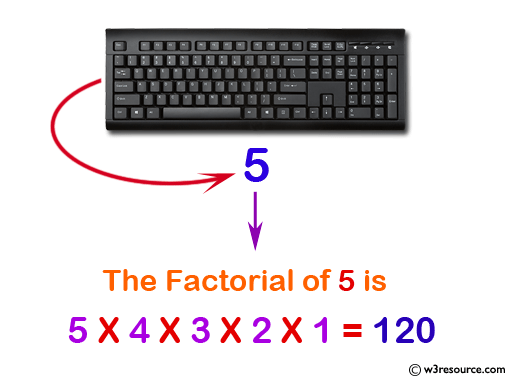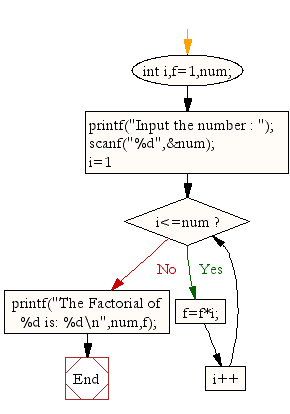﻿ C Program: Calculate the factorial of a given number - w3resource# C Exercises: Calculate the factorial of a given number

## C For Loop: Exercise-15 with Solution

Write a C program to calculate the factorial of a given number.

Pictorial Presentation:Sample Solution:

C Code:

``````#include <stdio.h>
void main(){
int i,f=1,num;

printf("Input the number : ");
scanf("%d",&num);

for(i=1;i<=num;i++)
f=f*i;

printf("The Factorial of %d is: %d\n",num,f);
}
```
```

Sample Output:

```Input the number : 5
The Factorial of 5 is: 120
```

Flowchart:C Programming Code Editor:

Improve this sample solution and post your code through Disqus.

What is the difficulty level of this exercise?

Test your Programming skills with w3resource's quiz.

﻿